# MCAT Physical : General Principles of Oxidation-Reduction

## Example Questions

### Example Question #1 : General Principles Of Oxidation Reduction

The Haber-Bosch process, or simply the Haber process, is a common industrial reaction that generates ammonia from nitrogen and hydrogen gas. A worker in a company generates ammonia from the Haber process. He then dissociates the gaseous ammonia in water to produce an aqueous solution. Since ammonia is a base, it will accept a proton from water, generating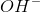and ammonium ion products. The two reactions involved are: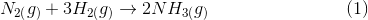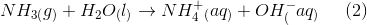A redox (reduction and oxidation) reaction involves a change in the oxidation state of atoms. Oxidation of an atom involves an increase in the oxidation state, whereas reduction involves a decrease in the oxidation state. A oxidized atom will usually become __________.

an anion because the number of electrons will be greater than the number of protons

a cation because the number of electrons will be greater than the number of protons

an anion because the number of electrons will be less than the number of protons

a cation because the number of electrons will be less than the number of protons

a cation because the number of electrons will be less than the number of protons

Explanation:

The question states that an oxidized atom will increase its oxidation state, which means that the atom will lose electrons and become more positively charged. Since the atom will lose electrons, the number of electrons will be less than the number of protons. Electrons are negatively charged and protons are positively charged; therefore, the presence of more protons in an oxidized atom will make the atom more positive. An oxidized atom will usually become a cation because its number of electrons will decrease.

Reduced atoms will gain electrons and become more negatively charged (decrease oxidation state). Notice that the reactions given in the passage are not redox reactions because the atoms in both reactions retain their oxidation states.

### Example Question #2 : General Principles Of Oxidation Reduction

Consider the following reaction.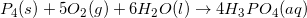Which of the following atoms is oxidized in the reaction?

Hydrogen

No atoms are oxidized in the reaction

Phosphorus

Oxygen

Phosphorus

Explanation:

The atom that is oxidized will have a higher oxidation number as a reactant than as a product. Since reactant phosphorus (P4) is in elemental form, it has an oxidation number of 0. As a product, phosphorus (PO43-) has an oxidation number of +5.

Total charge = (P) + 4(O) = –3

–3 = (P) + 4(–2) = (P) + (–8)

p = +5

Because it is now more positive, we say that the phosphorus has been oxidized.

### Example Question #3 : General Principles Of Oxidation Reduction

Consider the following reaction.What is the oxidizing reagent in the reaction?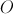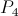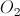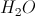Explanation:

The oxidizing reagent is the reactant, not the atom, that is responsible for receiving electrons from another atom in order to oxidize it. The oxygen gas goes from having an oxidation number of 0 to –2. This means that oxygen gas is reduced, but because it oxidized another atom by taking its electrons, we call it the oxidizing reagent.

Reactant oxygen is elemental, and has oxidation number 0.

Product oxygen is in H2O, with net charge of 0.

Total charge = 2(H) + (O) = 0

2(+1) + (O) = 2 + (O) = 0

O = –2

Decrease in oxidation number indicates reduction, and also identifies the oxidizing agent.

### Example Question #4 : General Principles Of Oxidation Reduction

Consider the following oxidation-reduction reaction.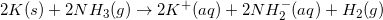Which of the following statements is true?

K is oxidized and K is the oxidizing reagent

K is reduced and K is the reducing reagent

K is reduced and NHis the oxidizing reagent

K is oxidized and NH3 is the oxidizing reagent

K is oxidized and NH3 is the oxidizing reagent

Explanation:

In the reaction, solid potassium (K) is initially in elemental form, so it has an oxidation state of 0. The potassium ions (K+) have a charge of +1 in the product. Since the potassium has lost an electron, it has been oxidized.

Since each hydrogen in reactants (NH3) has an oxidation state of +1, and the hydrogen gas (H2) has a charge of 0 as a product, the hydrogen has been reduced. Since it was reduced, we conclude that NH3 is the oxidizing reagent for the reaction.

### Example Question #5 : General Principles Of Oxidation Reduction

Consider the combustion of cyclohexane in air at 298K to give gaseous carbon dioxide and liquid water, as shown in this reaction.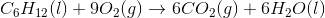In this reaction, __________ is the oxidizing agent and __________ is the reducing agent.

oxygen . . . carbon dioxide

cyclohexane . . . water

oxygen . . . cyclohexane

cyclohexane . . . oxygen

carbon dioxide . . . cyclohexane

oxygen . . . cyclohexane

Explanation:

In this reaction, the oxidation number of oxygen goes from, in diatomic gaseous oxygen, to, in both carbon dioxide and water. This indicates that it has gained electrons; a gain of electrons indicates that oxygen has been reduced. Since it is reduced, it is the oxidizing agent.

The oxidation number of carbon goes fromin cyclohexane to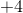in carbon dioxide. This indicates that it has lost electrons; a loss of electrons indicates that carbon has been oxidized. Since it is oxidized, cyclohexane is the reducing agent.

### Example Question #6 : General Principles Of Oxidation Reduction

Which compound is oxidized in the following reaction?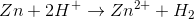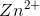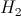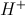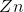Explanation:

Oxidation is defined by a loss of electrons, generally resulting in a more positive charge on an atom. Reduction is defined by a gain of electrons, generally resulting in a more negative charge on an atom. To identify the compound being oxidized, we need to find which compound is becoming more positive.Zinc is initially neutral, but gains a positive two charge as a product. Hydrogen initially has one positive charge, but becomes neutral in the product. The distinction becomes even clearer if we break the overall reaction into two half-reactions.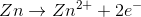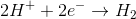We can see that zinc is losing electrons and hydrogen is gaining electrons. Zinc is thus being oxidized.

### Example Question #7 : General Principles Of Oxidation Reduction

Which of the following is an incorrect example of a combustion reaction?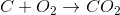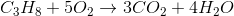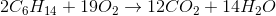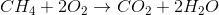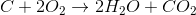Explanation:

We often hear of combustion reactions producing carbon dioxide and water. This is true when the reactants contain all three elements (hydrogen, oxygen, and carbon) needed to make carbon dioxide and water. The trick here is to understand that although combustion is just an oxidation reaction (ie, creating more carbon-oxygen bonds), all reactions must still be balanced. One answer choice fails to balance hydrogen in the reaction.Although we do see an oxidation reaction with the formation of carbon dioxide, the reaction is NOT balanced. This reaction cannot possibly take place as written.

### Example Question #8 : General Principles Of Oxidation Reduction

Which of the following is the oxidizing agent in the following equation?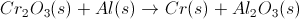Cr(s)

Al(s)

Cr3+

Al3+

Cr3+

Explanation:

An oxidizing agent is one that oxidizes another element and, becomes reduced in the process. Oxidizing and reducing agents are found in the reactants of the equation, eliminating Al3+ and Cr(s) as answer choices. Cr3+ gains three electrons to become Cr(s), which has an oxidation number of zero. Cr3+ becomes reduced, while Al(s) is oxidized, therefore, Cr3+ is the oxidizing agent. Remember "OIL RIG": Oxidation Is Loss of electrons and Reduction Is Gain of electrons.

### Example Question #9 : General Principles Of Oxidation Reduction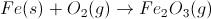In the above unbalanced redox reaction, which best describes the oxidation/reduction roles of iron and oxygen?

Iron is oxidized, oxygen is neither oxidized nor reduced

Iron is reduced, oxygen is oxidized

Iron is reduced, oxygen is neither oxidized nor reduced

Iron is oxidized, oxygen is reduced

Iron is reduced, oxygen is reduced

Iron is oxidized, oxygen is reduced

Explanation:

There are a couple options to solve this problem. First, we could simply remember that anything which combines with oxygen in a redox reaction is oxidized, and the associated oxygen is reduced.

Another option is to think about the oxidation numbers of each element (an element whose oxidation number increases is oxidized, and one whose oxidation number decreases is reduced). If we choose this method, recall that free elements (Fe and O2 in this case) have oxidation number 0. Also, oxygen in compounds has oxidation number -2. Oxygen's oxidation number decreases from 0 to -2, meaning that oxygen is reduced. Since oxidation and reduction occur at the same time in different elements of the redox reaction, iron's oxidation number must increase, so iron is oxidized.

### Example Question #10 : General Principles Of Oxidation Reduction

Consider the following combustion reaction.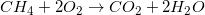Which of the following statements correctly describes carbon in the reaction?

Carbon is neither oxidized nor reduced in the reaction

Carbon is oxidized in the reaction

Carbon is the oxidizing agent in the reaction

Carbon is reduced in the reaction